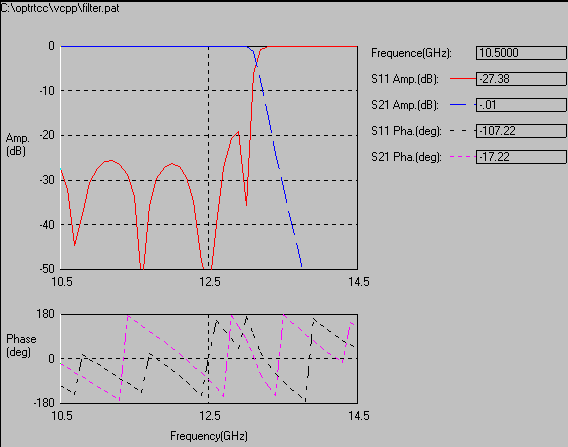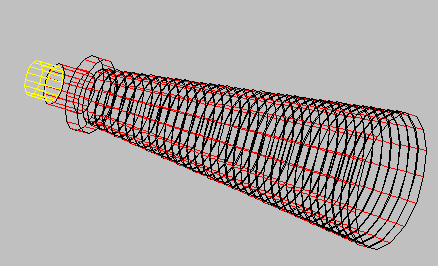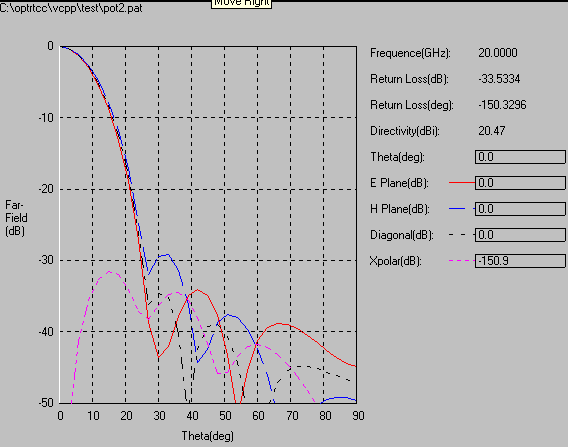# OPTRTCC EXAMPLES

## Examples of Optimisation of Mixed Rectangular and Circular Structures

### Example 1 Waveguide Filter

Example 1 is a low-pass filter of rectangular waveguide. The initial geometry (Figure 1) gives the response in frequency of Figure 2. After optimisation, the geometry was as shown in Figure 3 and the response as in Figure 4. The return loss over the band 10.7 to 12.75 GHz has improved from -24 dB to -40 dB while the level of rejection was maintained at 15 to 14.5 GHz.

### Example 2 Circular Horn

A circular multimode horn of large aperture was required to have a crosspolar level of -30 dB over a 10% bandwidth. The optimisation was carried out by varying the dimensions of the input geometry of the horn. Figure 5 shows the final geometry. Figure 6, Figure 7 and Figure 8 show radiation patterns at 19, 20 and 21 GHz where the peak cross-polarisation over the band is less than -32 dB.

### FIGURE 1 Initial Geometry### FIGURE 2 Initial Response### FIGURE 3 Final Geometry### FIGURE 4 Final Response### FIGURE 5 Optimised Geometry of Circular Horn### FIGURE 6 Radiation Pattern at 19 GHz### FIGURE 7 Radiation Pattern at 20 GHz### FIGURE 8 Radiation Pattern at 21 GHz